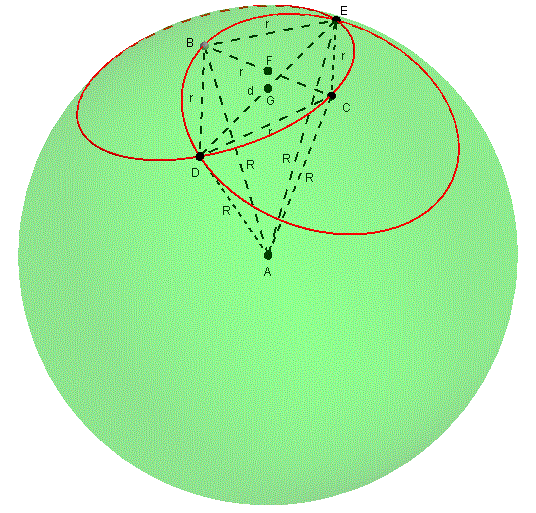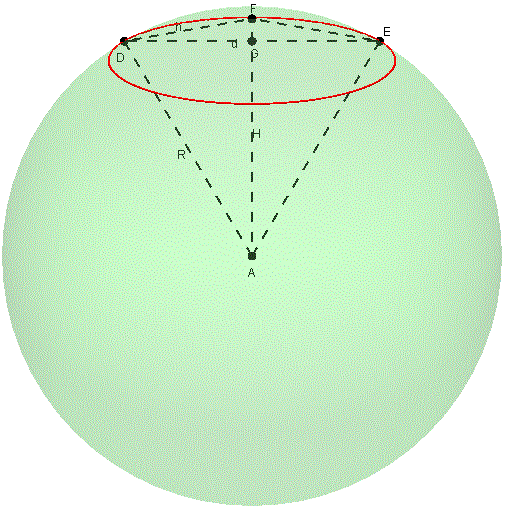# Finding the Radius of a Sphere

#### (An archive question of the week)

An interesting question came to us in 2016, where rather than using a well-known formula, it was necessary to work out both what data to use, and how to calculate the desired radius.

The question initially was vague:

Compass, Ruler, and Radius — of a Sphere

Using only a compass and a ruler, how can we calculate the radius of a hollow sphere?

Obviously it is very easy, but I don't know how to even start! I guess we draw some random circles of equal radius on the sphere surface, but then what? I don't know what to do.

I could think of many possible ways to do this, with various levels of accuracy, but I started with a mix of directness and humor:

Hi, Kyriakos.

I see nothing in the problem as stated that indicates it is "obviously very easy." What leads you to come to that conclusion?

I imagine there are many methods you could pursue, depending on the precision desired, the kind of compass, and what you are willing to do with it. For starters, some compasses can be opened out enough to be able to use them as calipers:

https://en.wikipedia.org/wiki/Calipers

(I don't suppose you could just use the point of the compass to threaten bodily harm to the person who made the sphere if he doesn't tell you its radius. "Lateral thinking" puzzles work something like this, what with joke answers for finding the height of a skyscraper using only a barometer, or some other unlikely tool.)

Finding the Radius of a Sphere

and of a tennis ball,

Radius of a Tennis Ball

In the latter, I suggested a way to do it using three rulers. But clearly something more specific is in view here. I continued:

My first serious thought involved using the compass to draw on the sphere: draw one circle on it with a known "radius" (a chord of the sphere); then put the compass point on that circle and draw another circle with the same "radius"; then use the compass to measure the straight-line distance between the two intersections. From that, you could in principle calculate the radius. But I don't want to work out a formula without knowing that's what I want to do.

This turns out to be the method I will be using; but the initial question is open enough to keep thinking. I might be missing something really easy; or there might be more to the rules of the game.

An easier way, though perhaps a little less exact in principle, is to measure the actual diameter of a drawn circle by putting the point on the circle and finding the greatest distance to a point on the other side of the circle. (This is something like the caliper idea, but doesn't require finding the exact opposite point on the sphere.) The "radius" is AB and the "diameter" is BD in the side view below:

***********
****           ****
*B**                   ****
**/|                         **
* / |                           *
* /  |                            *
* /r  |d/2                          *
* /    |                              *
*/     |                              *
*/      |                               *
A-------C           +                   *
*       |                               *
*      |                              *
*      |                              *
*     |                             *
*    |                            *
*   |                           *
** |                         **
*D**                   ****
****           ****
***********

From these measurements, there are several ways to calculate the radius R f the sphere, such as trigonometry or similar triangles.

If I had continued with this thought, I would have added two radii to the figure and used the Pythagorean theorem:

                   ***********
****           ****
*B**                   ****
**/|\                        **
* / |  \                        *
* /  |   \                        *
* /r  |d/2  \R                      *
* /    |       \                      *
*/     |        \                     *
*/      |          \                    *
A-------C-----------O                   *
*   h   |    R-h                        *
*      |                              *
*      |                              *
*     |                             *
*    |                            *
*   |                           *
** |                         **
*D**                   ****
****           ****
***********

In triangle ABC, we have (defining c = d/2 to avoid fractions) $$h^2 + c^2 = r^2$$, and in triangle OBC, $$(R-h)^2 + c^2 = R^2$$. Solving the first for h and substituting in the second to eliminate h, we end up with $$\displaystyle R = \frac{r^2}{2\sqrt{r^2 – c^2}}$$; replacing c with d/2 and simplifying, $$\displaystyle R = \frac{r^2}{\sqrt{4r^2 – d^2}}$$.

Pending more details from you, I think that leads to a decent answer, but I don't know that there isn't a much easier answer, perhaps even an "obvious" one. [One is to "rock" the ruler along the sphere to measure the arc length AB, then use that and r, but that requires numerical approximation rather than a formula.]

So, can you tell me where the question came from?

Many thanks for your reply!

To start with, I found this question on a Greek blog of math problems and riddles. I deduced it must be easy because several people had already claimed solutions. But they did not make these solutions visible, which only made my friends and me more curious.

If you can work further on the first method, the one with the two intersecting circles, I would be grateful!

For the other one, we must accept some approximation.

“Easy”, of course, is in the mind of the beholder.

And either method really requires approximation in practice; but the second does require a way to find opposite points on the circle, so although it might turn out to be just as accurate in practice, we are evidently looking for a theoretically precise answer. (I suspected there were also some hints as to the expected method, that I wasn’t privy to. Sometimes I will search for a problem across the web in hopes of finding the original wording, but this was in Greek so I couldn’t do that.)

I said that, but Kyriakos reported explicitly this time that my first method was what he wanted:

It seems that the two-circles method leads to a result that makes sense.

Draw two overlapping circles of the same radius r. (Compared to the sphere radius, this has to be relatively small). Construct a segment to connect the two points where these intersect. Call its length "L," and measure this with the ruler. Then the sphere radius is

(r/2)*sqrt{(4*r^2 - L^2)/((3*r^2 - L^2)}

But I cannot prove this result, so I would appreciate any assistance in explaining it.

My friend, who gave me the original question, received this solution (or rather "reply") from someone else. It did not come with any explanation; and furthermore, we don't even know if it is correct (only by intuition).

(Of course, you can’t really measure the segment directly with a ruler; he means to transfer the distance to a ruler using the compass.)

So I dove into the harder but more interesting method, now seeking to derive the reported formula:

I've been too busy to take the time to try to derive the formula until today, but this morning I managed it in perhaps ten minutes of free time. Then I had to write it up carefully....

Here is a picture:We have a sphere with radius R, center A, and two circles with radius r, centers B and C, that intersect at D and E. The distance DE is d. We want first to express d in terms of R and r, and then to solve for R.

First consider isosceles triangle ABC, with legs R and base r. We conclude that the altitude of this triangle, from A to the midpoint of BC, is

H = sqrt(R^2 - (r/2)^2)
= sqrt(4R^2 - r^2)/2

For clarity, r is not really the radius of the circles (whose centers are inside the sphere), but the length of a chord from the “center” B or C to any point on the circumference. Likewise, DE is a straight line distance measured through the sphere. F is the midpoint of BC, and G is the midpoint of DE, both in the interior. Distance H is |AF| in the figure. I used GeoGebra to make the figure.

In the next figure we have a cross-section through ADE; as seen here, the two circles look like the same ellipse, and B and C on the sphere coincide with F. But all the lines shown are coplanar. We first look at triangle ADF (which is not isosceles):

Now consider the plane of triangle ADE:In triangle ADF, DF is the altitude of the equilateral triangle BCD, h = r sqrt(3)/2, and AF = H = sqrt(4R^2 - r^2)/2.

By the law of cosines,

cos(DAF) = [R^2 + H^2 - h^2]/[2RH]
= [R^2 + (4R^2 - r^2)/4 - 3(r^2)/4]/[2R sqrt(4R^2 - r^2)/2]
= [R^2 + R^2 - (r^2)/4 - 3(r^2)/4]/[R sqrt(4R^2 - r^2)]
= [2R^2 - r^2]/[R sqrt(4R^2 - r^2)]


Next, we look at triangle ADG, which shares the angle DAF, and then use the previously determined cosine to find an expression for d:

In right triangle ADG, where G is the midpoint of DE, we have

sin(DAF) = (d/2)/R

Now,

d = 2R sin(DAF)
= 2R sqrt(1 - cos^2(DAF))
= 2R sqrt(1 - [2R^2 - r^2]^2/[R sqrt(4R^2 - r^2)]^2)
= 2R sqrt(1 - [2R^2 - r^2]^2/[R^2 (4R^2 - r^2)])
= 2R sqrt(R^2 (4R^2 - r^2) - [2R^2 - r^2]^2]/[R^2 (4R^2 - r^2)])
= 2R sqrt([4R^4 - r^2R^2 - 4R^4 + 4r^2R^2 - r^4]/[4R^4 - r^2R^2])
= 2R sqrt([3r^2R^2 - r^4]/[4R^4 - r^2R^2])
= 2 sqrt([3r^2R^2 - r^4]/[4R^2 - r^2])

We have now expressed d in terms of r and R; now we solve for R, as planned:

Solving this for R,

d^2 = 4[3r^2R^2 - r^4]/[4R^2 - r^2]
d^2 [4R^2 - r^2] = 4[3r^2R^2 - r^4]
4d^2R^2 - d^2r^2 = 12r^2R^2 - 4r^4
4d^2R^2 - 12r^2R^2 = d^2r^2 - 4r^4
[4d^2 - 12r^2]R^2 = d^2r^2 - 4r^4
R^2 = [d^2r^2 - 4r^4]/[4d^2 - 12r^2]
= r^2[d^2 - 4r^2]/[4(d^2 - 3r^2)]

R = r/2 sqrt([d^2 - 4r^2]/[d^2 - 3r^2])
= r/2 sqrt([4r^2 - d^2]/[3r^2 - d^2])

And that's your formula.

Formatted nicely, the formula is $$\displaystyle R = \frac{r}{2} \sqrt{\frac{4r^2 – d^2}{3r^2 – d^2}}$$.

I’ve played with this and see no nice, “obvious” geometrical meaning for it. But one thing we can do to check it out is to try some special cases.

First, what if the sphere is really a plane (that is, the radius in “infinite”)? Then we just have the familiar figure of two overlapping circles in a plane, and can easily calculate that $$d = r\sqrt{3}$$ (twice the altitude of an equilateral triangle). Putting that into the formula, $$\displaystyle R = \frac{r}{2} \sqrt{\frac{4r^2 – 3r^2}{3r^2 – 3r^2}} = \frac{r}{2} \sqrt{\frac{r^2}{0}}$$ which is undefined as expected.

Second, what if each circle were a great circle? Then $$r = R\sqrt{2}$$ and $$d = 2R$$. Putting those into the formula, we get $$\displaystyle R = \frac{R\sqrt{2}}{2} \sqrt{\frac{8R^2 – 4R^2}{6R^2 – 4R^2}} = \frac{R\sqrt{2}}{2} \sqrt{\frac{4R^2}{2R^2}} = \frac{R\sqrt{2}}{2} \sqrt{2} = R$$ as expected.

This site uses Akismet to reduce spam. Learn how your comment data is processed.